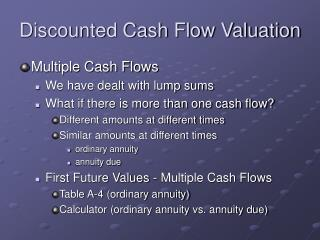DownloadDownload PresentationDiscounted Cash Flow Valuation

# Discounted Cash Flow Valuation

Download Presentation## Discounted Cash Flow Valuation

- - - - - - - - - - - - - - - - - - - - - - - - - - - E N D - - - - - - - - - - - - - - - - - - - - - - - - - - -
##### Presentation Transcript

1. Discounted Cash Flow Valuation • Multiple Cash Flows • We have dealt with lump sums • What if there is more than one cash flow? • Different amounts at different times • Similar amounts at different times • ordinary annuity • annuity due • First Future Values - Multiple Cash Flows • Table A-4 (ordinary annuity) • Calculator (ordinary annuity vs. annuity due)

2. Discounted Cash Flow Valuation • How the cash flows can be viewed • Series of lump sums • Series of payments • Some examples that are standard ordinary annuities for Future Value Calculations • Savings • Retirement • Some ordinary annuities with Present Value • car loans • mortgages

3. Discounted Cash Flow Valuation • Back to the basics of the equation with annuities: • PV = PMT x (PVIFA, n, k) where n is number of payments and k is the interest rate for the period • FV = PMT x (FVIFA, n, k) • PMT = PV / (PVIFA, n, k) • Remember must know all on the right side... • What about n and k?

4. Discounted Cash Flow Valuation • Formula for PVIFA • PVIFA = {1 - (1/ [1 + k])n }/ k • Text has PVIFA = ∑ 1/(1 + k)n • These are the same! • Formula for FVIFA • FVIFA = {[1 + k])n - 1}/ k • Text has FVIFA = ∑ (1 + k)n-1 • These are the same! • Add these two formulas and now you can “solve” for k or n as well. • Find FVIFA in Problem 17

5. Three Methods for Annuities • Table in back of the book… • PV = Payment x PVIFA (n, k) • Formula • PV = Payment x {1 - (1/ [1 + k])n }/ k • Calculator • TVM keys • Example – Problem 18

6. Discounted Cash Flow Valuation • Using the calculator for k (I) or n (N) • some tricks to remember… • direction of the cash flow is important for determining the k or n…usually PV is negative • be sure and have payments per year correct and corresponding to compound per year…these are the P/Y and C/Y functions • Example • \$1,000 PMT, -\$6710 PV, N=10 (P/Y and C/Y =1) • Compute I…answer is 8%

7. Discounted Cash Flow Valuation • Perpetuities • The never ending interest payment... • Special formula from limits in math • Problem 23

8. Discounted Cash Flow Valuation • Amortization • Paying off loans • lump sum payoff (pure discount) • interest only and principal at the end… • equal payment series (interest and some principal at each payment) • Amortization Schedule • the principal reduction schedule • typical ordinary annuity problem… • calculator on the remaining balance

9. Amortization • Interest is accumulated based on the outstanding principal • Think of a loan where each period you pay it off and then “re-borrow” the reduced principal • Table for Amortization • Find payment first • Beginning balance x interest rate • Portion of Payment to interest, remainder to principal • Remaining principal is Ending Balance • Start again next period • Problem 42 annual payments and monthly • Via Calculator for Problem 42